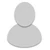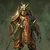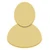## FANDOM

4,625 Pages

One way to increase the effectiveness of your Familiars is through Evolution.

Evolution allows you to combine two Familiars of the same evolutionary line to increase the stats, and rarity of a Familiar. Evolving a 1* Familiar two or more times will change the Familiar's rarity, and sometimes, its skill.

## Familiar Evolution

 “ By combining two of the same familiar, it is possible to create an evolved familiar that is stronger than the originals, with a different appearance. The rarity of some familiars may change after evolving, and it's even possible their initial stats may be lower. Rest assured that after gaining some levels their new stats will surpass the old. ” — In-game Help

### How to Evolve

 “ Tap a familiar to select or deselect it. Tap and hold on a familiar to view it's detailed stats. First choose the target familiar, or "spawn," then choose the seed. Gold is necessary to complete the evolution. Note that familiars currently in an adventuring party may not be selected. ” — In-game Help

### Multiple Evolutions

 “ A familiar can only evolve a limited number of times--the more star icons it has, the more it can evolve. Evolving twice increases the familiar's rarity and changes it's skills. ” — In-game Help

### Spawn

The base Familiar to be evolved. The Spawn absorbs 5% of the Seed's stats (unless the seed is at max level in which case the Spawn absorbs 10%). The Resulting Familiar will have increased stats (including empowered bonuses), a new look, name, and sometimes skill.

### Seed

The familiar used as raw material in an evolution is called the "seed." Once used, the seed is removed from your familiars. Only one seed may be chosen in each evolution.

The new evolved familiar will inherit 5% of the stats (including empower bonuses) for non-max level seeds and 10% (including empower bonuses) from max-level seeds.

## Evolution Glossary

The most common evolution terms/acronyms from best to worst:

• POPE = Perfect Over Perfect Evo: Mostly referred as short form of OPE400. 8x1* fully leveled + a total of 400 crystals on the appropriate spawns. With 2-star familiars, it carries the meaning of OPE100.
• Note: POPE was originated from failed autocorrect of mobile devices and it is widely accepted as an alternative of OPE400 / OPE100.
• OPE xx = Over Perfect Evo: 8x1* fully leveled + a total of xx crystals on the appropriate spawns. An OPE 100 would have 100 crystals. See the Tutorial: Making an OPE page for more information.
• PE = Perfect Evo: 8x1* fully leveled, no crystals.
• NPE = Near Perfect Evo: Below, but approaching, PE Stats. See Evolution Combinations below.
• Ghetto: A familiar that has stats very far from PE, most easily achieved by evolving with seeds below max level. Pejorative term.
• Pact: A familiar that was obtained from a pact and was not evolved in any way.

## Formula

Either of these formulas work. The Pact Max Stats is equal to the Pact Base Stats Plus the Level Boosts.

```  The Resultant Familiar's Base Level 1 Stats
+ Level Boosts
+ Seed Bonus
+ Spawn's Seed Bonus
+ Spawn's Spawn's Seed Bonus```

```  The Resultant Familiar's Max Level Pact Stats
+ Seed Bonus
+ Spawn's Seed Bonus
+ Spawn's Spawn's Seed Bonus
```

### Example: Unbound Gargoyle II

• Gargoyle has a Max HP of 4,680 (468 Seed Bonus).
• Gargoyle II PE has a Base HP of 2,360 + 3,260 (70 Levels of Stat Boost) + 468 (Seed Bonus) = 6,088 (609 Seed Bonus). Gargoyle II's Spawn does not have a seed, nor does his Spawn's Spawn, so they are ignored.
• Unbound Gargoyle has a Base HP of 3,120 + 4,160 (Levels) + 609 (Seed Bonus) + 468 (Spawn's Seed) = 8,357 (836 Seed Bonus). Unbound Gargoyle does not get the "Spawn's Spawn's Seed Bonus" since his evolution does not go back that far.
• Unbound Gargoyle II has a Base HP of 3,590 + 5,150 (Levels) + 836 (Seed) + 609 (Spawn's Seed) + 468 (Spawn's Spawn's Seed) = 10,653.

The Spawn's Seed and Spawn's Spawn's Seed become critical when dealing with no PE evolutions. For example, in a 7 Fam evolution, one Rank 3 will not have a Rank 1 Seed Bonus applied. It's important when calculating those stats to know who doesn't get the bonus.

This picture shows the Seed's that shape the Rank 4 Familiar's Stats:

The Rank 3 Familiar's Seed bonuses use the same pattern, only they have 2 Seed bonuses applied instead of 3.

## Tips & Tricks

The following information has been obtained through trial and error, and some guesswork.

After the evolution, the familiar may CHANGE SKILLS. The Spawn also retains its level, however when upgrading in rarity (which includes an increase in level cap), this level will change depending on rarity. Common Familiars have a max level of 30, Uncommon familiars have 40, Rare ones 70, and Epics and Legendaries 99. Evolution resulting in increased rarity will raise the Spawn's max level. The Spawn's skill level will also be kept during evolution.Crossbowman Evolution, Spawn with Skill To illustrate the point of Skill level inheritance, take a look at this Crossbowman.Wight Knight Evolution, Skilled Seed. To further illustrate Skill Level inheritance the more skilled Wight Knight was placed as a seed.Crossbowman II to Sureshot Evolution. When the Skill level changes (a result with an odd number of stars), the Skill Level experience is still inherited from the Spawn. Due to some skills costing more expereince to level, the familiars Skill Level may change.
Both the Spawn and Seed can be at Max Lvl before evolving them. However the Spawns level does not impact the evolved familiars stats not does it reset upon evolution, the Seed should be at Max Lvl in order to qualify for the 10% stat inheritance to the Evolved. Any stats increased by crystals on the seed will transfer based on the full value, meaning with 10 crystals on a stat you will transfer an additional 50 points. Getting a Two Star unit as a seed is less ideal than two One Star units that have been evolved at max level. This means to get the optimal stats for a final 4 Star Unit you will require eight 1 star units of that type. Each of those have to be leveled to their max before they are used as a Seed in the evolution.

## Evolution Combinations

Two tools are available in our Blood Brothers Database to assist you in Evolutions: 

1. EvoCalculator v2 - Select the name of a 1* familiar and it will tell you the Base, Pact, and Perfect Evolved Max stats of that familiar and all evolutions related to it. It will also give you the PE stats and Percent of Perfect for all possible non-perfect evolutions.

2. LevelCalc - Select the exact familiar you wish to evaluate. Enter the stats you see for your familiars (say you want to check on one in the Bazaar). Enter the Level of that Familiar. The calculator will return the Perfect Evolved Stats for that fam, how many points above or below perfect your fam is, and even tell you what his/her stats will be at max level and how far from perfect (Percentage) it will be.

Another evolution guide, with all possible combinations, is also available here: 

### Evolution Table

You will find below the best combination for different non-perfect evolutions (NPE of 2, 3, 4, 5, 6, and 7 Familiars).

Evolution
Cards NPE % Steps
1 Card 1x EP4 ~82.00% EP4
2 Cards 1x R1 1x EP3 ~86.97% EP3 + R1
1x R2 1x EP3 ~87.15% EP3 + R2
2x EP3 ~88.52% EP3 + EP3
3 Cards 2x R1 1x R2 ~90.79% (R2 + R1) + R1
1x R1 2x R2 ~91.69% (R2 + R2) + R1
3x R2 ~92.60% (R2 + R2) + R2
1x R1 1x R2 1x EP3 ~93.05% (R2 + R1) + EP3
2x R2 1x EP3 ~93.96% (R2 + R2) + EP3
4 Cards 1x R1 3x R2 ~94.42% (R2 + R2) + (R2 + R1)
4x R2 ~94.51% (R2 + R2) + (R2 + R2)
4x R1 ~95.32% [(R1 + R1) + R1] + R1
3x R1 1x R2 ~96.23% [(R1 + R1) + R1] + R2
3x R1 1x EP3 ~97.59% [(R1 + R1) + R1] + EP3
2x R1 2x R2 ~97.14% [(R1 + R1) + R2] + R2
2x R1 1x R2 1x EP3 ~98.50% [(R1 + R1) + R2] + EP3
5 Cards 5x R1 ~96.69% [(R1 + R1) + (R1 + R1)] + R1
4x R1 1x R2 ~98.05% [(R1 + R1) + R1] + (R2 + R1)
4x R1 1x EP3 ~98.96% [(R1 + R1) + (R1 + R1)] + EP3
3x R1 2x R2 ~98.96% [(R1 + R1) + R2] + (R2 + R1)
2x R1 3x R2 ~99.05% [(R1 + R1) + R2] + (R2 + R2)
6 Cards 6x R1 ~98.50% [(R1 + R1) + R1] + [(R1 + R1) + R1]
5x R1 1x R2 ~99.41% [(R1 + R1) + (R1 + R1)] + (R2 + R1)
4x R1 2x R2 ~99.50% [(R1 + R1) + (R1 + R1)] + (R2 + R2)
7 Cards 6x R1 1x R2 ~99.95% [(R1 + R1) + (R1 + R1)] + [(R1 + R1) + R2]
7x R1 ~99.86% [(R1 + R1) + (R1 + R1)] + [(R1 + R1) + R1]
8 Cards 8x R1 100% (PE) [(R1 + R1) + (R1 + R1)] + [(R1 + R1) + (R1 + R1)]

### 4-Familiar Evolution

Here's an example of an evolution featuring 4 Familiars: 4 Rare 1*. Formula: (((1+1)+1)+1). Assuming that all the familiars are evolved at Max level, this combination is around 95% of PE.

Here's another example of an evolution featuring 4 Familiars: 2 Rare 1*, 1 Rare 2* and 1 Epic 3*. Formula: (((1+1)+2)+3). Assuming that all the familiars are evolved at Max level, this combination is around 98.5% of PE.

### 5-Familiar Evolution

Here's an example of an evolution featuring 5 Familiars: 2 Rare 1* and 3 Rare 2*. Formula: (((1+1)+2)+(2+2)). Assuming that all the familiars are evolved at Max level, this combination is around 99.1% of PE.

### 6-Familiar Evolution

Here's an example of an evolution featuring 6 Familiars: 4 Rare 1* and 2 Rare 2*. Formula: (((1+1)+(1+1))+(2+2)). Assuming that all the familiars are evolved at Max level, this combination is around 99.5% of PE.

### 7-Familiar Evolution

Here's an example of an evolution featuring 7 Familiars: 6 Rare 1* and 1 Rare 2*. Formula: (((1+1)+(1+1))+((1+1)+2)). Assuming that all the familiars are evolved at Max level, this combination is around 99.9% of PE.

## Evolved vs. Cemetery/Captured Familiars

A 2-star familiar that is created by evolving two 1-starred familiars (even if both are level 1) will be 10-20% stronger than the same familiar who is received either by capturing it or getting it from a pact. The same applies for 3 and 4 starred familiars.Most familiars that were obtained through capture or drawn from a pact will end in 0 for each stat. Some familiars with the growth type 'Late Bloomer' may be exceptions to this rule, but most familiars with Fast or Normal growth will end with 0 in each stat.

## Start a Discussion Discussions about Evolution

•#### Evolution!

4 messages
•Thanks so much, thats what i had in mind i i failed because i lved his proc up when the acual spawn had to be lved :( oh well ... but again tha...
•Oh, darn. :( Although you might be fine as long as the seeds were always at max level (spawn can be levelled after evolution with no effect...
•#### Question about 6x cards NPE (99.50%).

8 messages
•@ Elderberries, Yes, the two are identical. '''[(R1 + R1) + (R1 + R1)] + (R2 + R2)''' Taking the above case,...
•i agree with optimus. There is no point building a PE fam.The [(R1+R1)+R2]+EP3 i think its the best compination. And for me POPE is j...